# Quick start guide

In the following we provide some pointers about which functions and classes to use for different problems related to optimal transport (OT) and machine learning. We refer when we can to concrete examples in the documentation that are also available as notebooks on the POT Github.

Note

For a good introduction to numerical optimal transport we refer the reader to the book by Peyré and Cuturi 15. For more detailed introduction to OT and how it can be used in ML applications we refer the reader to the following OTML tutorial.

Note

Since version 0.8, POT provides a backend to automatically solve some OT problems independently from the toolbox used by the user (numpy/torch/jax). We provide a discussion about which functions are compatible in section Backend section .

## Why Optimal Transport ?

### When to use OT

Optimal Transport (OT) is a mathematical problem introduced by Gaspard Monge in 1781 that aim at finding the most efficient way to move mass between distributions. The cost of moving a unit of mass between two positions is called the ground cost and the objective is to minimize the overall cost of moving one mass distribution onto another one. The optimization problem can be expressed for two distributions $$\mu_s$$ and $$\mu_t$$ as

$\min_{m, m \# \mu_s = \mu_t} \int c(x,m(x))d\mu_s(x) ,$

where $$c(\cdot,\cdot)$$ is the ground cost and the constraint $$m \# \mu_s = \mu_t$$ ensures that $$\mu_s$$ is completely transported to $$\mu_t$$. This problem is particularly difficult to solve because of this constraint and has been replaced in practice (on discrete distributions) by a linear program easier to solve. It corresponds to the Kantorovitch formulation where the Monge mapping $$m$$ is replaced by a joint distribution (OT matrix expressed in the next section) (see Solving optimal transport).

From the optimization problem above we can see that there are two main aspects to the OT solution that can be used in practical applications:

• The optimal value (Wasserstein distance): Measures similarity between distributions.

• The optimal mapping (Monge mapping, OT matrix): Finds correspondences between distributions.

In the first case, OT can be used to measure similarity between distributions (or datasets), in this case the Wasserstein distance (the optimal value of the problem) is used. In the second case one can be interested in the way the mass is moved between the distributions (the mapping). This mapping can then be used to transfer knowledge between distributions.

#### Wasserstein distance between distributions

OT is often used to measure similarity between distributions, especially when they do not share the same support. When the support between the distributions is disjoint OT-based Wasserstein distances compare favorably to popular f-divergences including the popular Kullback-Leibler, Jensen-Shannon divergences, and the Total Variation distance. What is particularly interesting for data science applications is that one can compute meaningful sub-gradients of the Wasserstein distance. For these reasons it became a very efficient tool for machine learning applications that need to measure and optimize similarity between empirical distributions.

Numerous contributions make use of this an approach is the machine learning (ML) literature. For example OT was used for training Generative Adversarial Networks (GANs) in order to overcome the vanishing gradient problem. It has also been used to find discriminant or robust subspaces for a dataset. The Wasserstein distance has also been used to measure similarity between word embeddings of documents or between signals or spectra.

#### OT for mapping estimation

A very interesting aspect of OT problem is the OT mapping in itself. When computing optimal transport between discrete distributions one output is the OT matrix that will provide you with correspondences between the samples in each distributions.

This correspondence is estimated with respect to the OT criterion and is found in a non-supervised way, which makes it very interesting on problems of transfer between datasets. It has been used to perform color transfer between images or in the context of domain adaptation. More recent applications include the use of extension of OT (Gromov-Wasserstein) to find correspondences between languages in word embeddings.

### When to use POT

The main objective of POT is to provide OT solvers for the rapidly growing area of OT in the context of machine learning. To this end we implement a number of solvers that have been proposed in research papers. Doing so we aim to promote reproducible research and foster novel developments.

One very important aspect of POT is its ability to be easily extended. For instance we provide a very generic OT solver ot.optim.cg that can solve OT problems with any smooth/continuous regularization term making it particularly practical for research purpose. Note that this generic solver has been used to solve both graph Laplacian regularization OT and Gromov Wasserstein 30.

Note

POT is originally designed to solve OT problems with Numpy interface and is not yet compatible with Pytorch API. We are currently working on a torch submodule that will provide OT solvers and losses for the most common deep learning configurations.

#### When not to use POT

While POT has to the best of our knowledge one of the most efficient exact OT solvers, it has not been designed to handle large scale OT problems. For instance the memory cost for an OT problem is always $$\mathcal{O}(n^2)$$ in memory because the cost matrix has to be computed. The exact solver in of time complexity $$\mathcal{O}(n^3\log(n))$$ and the Sinkhorn solver has been proven to be nearly $$\mathcal{O}(n^2)$$ which is still too complex for very large scale solvers.

If you need to solve OT with large number of samples, we recommend to use entropic regularization and memory efficient implementation of Sinkhorn as proposed in GeomLoss. This implementation is compatible with Pytorch and can handle large number of samples. Another approach to estimate the Wasserstein distance for very large number of sample is to use the trick from Wasserstein GAN that solves the problem in the dual with a neural network estimating the dual variable. Note that in this case you are only solving an approximation of the Wasserstein distance because the 1-Lipschitz constraint on the dual cannot be enforced exactly (approximated through filter thresholding or regularization). Finally note that in order to avoid solving large scale OT problems, a number of recent approached minimized the expected Wasserstein distance on minibtaches that is different from the Wasserstein but has better computational and statistical properties.

## Optimal transport and Wasserstein distance

Note

In POT, most functions that solve OT or regularized OT problems have two versions that return the OT matrix or the value of the optimal solution. For instance ot.emd returns the OT matrix and ot.emd2 returns the Wassertsein distance. This approach has been implemented in practice for all solvers that return an OT matrix (even Gromov-Wasserstsein).

### Solving optimal transport

The optimal transport problem between discrete distributions is often expressed as

\begin{align}\begin{aligned}\gamma^* = arg\min_{\gamma \in \mathbb{R}_+^{m\times n}} \quad \sum_{i,j}\gamma_{i,j}M_{i,j}\\s.t. \gamma 1 = a; \gamma^T 1= b; \gamma\geq 0\end{aligned}\end{align}

where:

• $$M\in\mathbb{R}_+^{m\times n}$$ is the metric cost matrix defining the cost to move mass from bin $$a_i$$ to bin $$b_j$$.

• $$a$$ and $$b$$ are histograms on the simplex (positive, sum to 1) that represent the weights of each samples in the source an target distributions.

Solving the linear program above can be done using the function ot.emd that will return the optimal transport matrix $$\gamma^*$$:

# a and b are 1D histograms (sum to 1 and positive)
# M is the ground cost matrix
T = ot.emd(a, b, M)  # exact linear program


The method implemented for solving the OT problem is the network simplex. It is implemented in C from 1. It has a complexity of $$O(n^3)$$ but the solver is quite efficient and uses sparsity of the solution.

#### Examples of use for ot.emd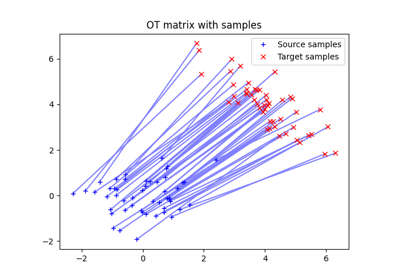Optimal Transport between 2D empirical distributions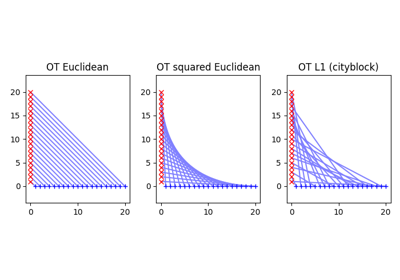Optimal Transport with different gournd metrics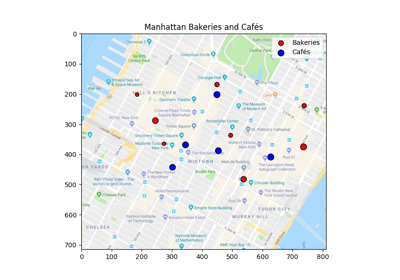Introduction to Optimal Transport with Python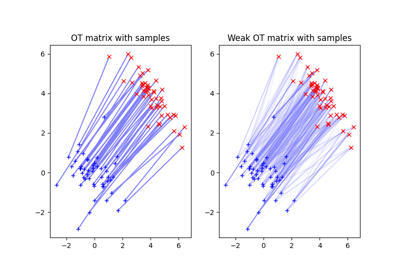Weak Optimal Transport VS exact Optimal Transport

### Computing Wasserstein distance

The value of the OT solution is often more interesting than the OT matrix:

\begin{align}\begin{aligned}OT(a,b) = \min_{\gamma \in \mathbb{R}_+^{m\times n}} \quad \sum_{i,j}\gamma_{i,j}M_{i,j}\\s.t. \gamma 1 = a; \gamma^T 1= b; \gamma\geq 0\end{aligned}\end{align}

It can computed from an already estimated OT matrix with np.sum(T*M) or directly with the function ot.emd2.

# a and b are 1D histograms (sum to 1 and positive)
# M is the ground cost matrix
W = ot.emd2(a, b, M)  # Wasserstein distance / EMD value


Note that the well known Wasserstein distance between distributions a and b is defined as

\begin{align}\begin{aligned}W_p(a,b)=(\min_{\gamma \in \mathbb{R}_+^{m\times n}} \sum_{i,j}\gamma_{i,j}\|x_i-y_j\|_p)^\frac{1}{p}\\s.t. \gamma 1 = a; \gamma^T 1= b; \gamma\geq 0\end{aligned}\end{align}

This means that if you want to compute the $$W_2$$ you need to compute the square root of ot.emd2 when providing M = ot.dist(xs, xt), that uses the squared euclidean distance by default. Computing the $$W_1$$ Wasserstein distance can be done directly with ot.emd2 when providing M = ot.dist(xs, xt, metric='euclidean') to use the Euclidean distance.

#### Examples of use for ot.emd2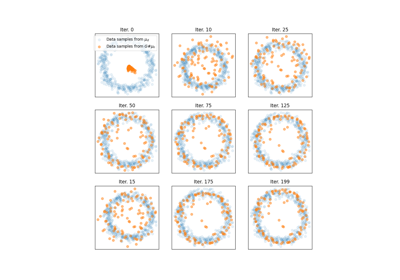Wasserstein 2 Minibatch GAN with PyTorch

### Special cases

Note that the OT problem and the corresponding Wasserstein distance can in some special cases be computed very efficiently.

For instance when the samples are in 1D, then the OT problem can be solved in $$O(n\log(n))$$ by using a simple sorting. In this case we provide the function ot.emd_1d and ot.emd2_1d to return respectively the OT matrix and value. Note that since the solution is very sparse the sparse parameter of ot.emd_1d allows for solving and returning the solution for very large problems. Note that in order to compute directly the $$W_p$$ Wasserstein distance in 1D we provide the function ot.wasserstein_1d that takes p as a parameter.

Another special case for estimating OT and Monge mapping is between Gaussian distributions. In this case there exists a close form solution given in Remark 2.29 in 15 and the Monge mapping is an affine function and can be also computed from the covariances and means of the source and target distributions. In the case when the finite sample dataset is supposed Gaussian, we provide ot.da.OT_mapping_linear that returns the parameters for the Monge mapping.

## Regularized Optimal Transport

Recent developments have shown the interest of regularized OT both in terms of computational and statistical properties. We address in this section the regularized OT problems that can be expressed as

\begin{align}\begin{aligned}\gamma^* = arg\min_{\gamma \in \mathbb{R}_+^{m\times n}} \quad \sum_{i,j}\gamma_{i,j}M_{i,j} + \lambda\Omega(\gamma)\\ s.t. \gamma 1 = a; \gamma^T 1= b; \gamma\geq 0\end{aligned}\end{align}

where :

• $$M\in\mathbb{R}_+^{m\times n}$$ is the metric cost matrix defining the cost to move mass from bin $$a_i$$ to bin $$b_j$$.

• $$a$$ and $$b$$ are histograms (positive, sum to 1) that represent the weights of each samples in the source an target distributions.

• $$\Omega$$ is the regularization term.

We discuss in the following specific algorithms that can be used depending on the regularization term.

### Entropic regularized OT

This is the most common regularization used for optimal transport. It has been proposed in the ML community by Marco Cuturi in his seminal paper 2. This regularization has the following expression

$\Omega(\gamma)=\sum_{i,j}\gamma_{i,j}\log(\gamma_{i,j})$

The use of the regularization term above in the optimization problem has a very strong impact. First it makes the problem smooth which leads to new optimization procedures such as the well known Sinkhorn algorithm 2 or L-BFGS (see ot.smooth ). Next it makes the problem strictly convex meaning that there will be a unique solution. Finally the solution of the resulting optimization problem can be expressed as:

$\gamma_\lambda^*=\text{diag}(u)K\text{diag}(v)$

where $$u$$ and $$v$$ are vectors and $$K=\exp(-M/\lambda)$$ where the $$\exp$$ is taken component-wise. In order to solve the optimization problem, one can use an alternative projection algorithm called Sinkhorn-Knopp that can be very efficient for large values of regularization.

The Sinkhorn-Knopp algorithm is implemented in ot.sinkhorn and ot.sinkhorn2 that return respectively the OT matrix and the value of the linear term. Note that the regularization parameter $$\lambda$$ in the equation above is given to those functions with the parameter reg.

>>> import ot
>>> a = [.5, .5]
>>> b = [.5, .5]
>>> M = [[0., 1.], [1., 0.]]
>>> ot.sinkhorn(a, b, M, 1)
array([[ 0.36552929,  0.13447071],
[ 0.13447071,  0.36552929]])


More details about the algorithms used are given in the following note.

Note

The main function to solve entropic regularized OT is ot.sinkhorn. This function is a wrapper and the parameter method allows you to select the actual algorithm used to solve the problem:

In addition to all those variants of Sinkhorn, we have another implementation solving the problem in the smooth dual or semi-dual in ot.smooth. This solver uses the scipy.optimize.minimize function to solve the smooth problem with L-BFGS-B algorithm. Tu use this solver, use functions ot.smooth.smooth_ot_dual or ot.smooth.smooth_ot_semi_dual with parameter reg_type='kl' to choose entropic/Kullbach Leibler regularization.

Choosing a Sinkhorn solver

By default and when using a regularization parameter that is not too small the default Sinkhorn solver should be enough. If you need to use a small regularization to get sharper OT matrices, you should use the ot.bregman.sinkhorn_stabilized solver that will avoid numerical errors. This last solver can be very slow in practice and might not even converge to a reasonable OT matrix in a finite time. This is why ot.bregman.sinkhorn_epsilon_scaling that relie on iterating the value of the regularization (and using warm start) sometimes leads to better solutions. Note that the greedy version of the Sinkhorn ot.bregman.greenkhorn can also lead to a speedup and the screening version of the Sinkhorn ot.bregman.screenkhorn aim a providing a fast approximation of the Sinkhorn problem. For use of GPU and gradient computation with small number of iterations we strongly recommend the ot.bregman.sinkhorn_log solver that will no need to check for numerical problems.

Recently Genevay et al. 23 introduced the Sinkhorn divergence that build from entropic regularization to compute fast and differentiable geometric divergence between empirical distributions. Note that we provide a function that computes directly (with no need to precompute the M matrix) the Sinkhorn divergence for empirical distributions in ot.bregman.empirical_sinkhorn_divergence. Similarly one can compute the OT matrix and loss for empirical distributions with respectively ot.bregman.empirical_sinkhorn and ot.bregman.empirical_sinkhorn2.

Finally note that we also provide in ot.stochastic several implementation of stochastic solvers for entropic regularized OT 18 19. Those pure Python implementations are not optimized for speed but provide a robust implementation of algorithms in 18 19.

#### Examples of use for ot.sinkhornOptimal Transport between 2D empirical distributionsIntroduction to Optimal Transport with Python

### Other regularizations

While entropic OT is the most common and favored in practice, there exists other kinds of regularizations. We provide in POT two specific solvers for other regularization terms, namely quadratic regularization and group Lasso regularization. But we also provide in ot.optim two generic solvers that allows solving any smooth regularization in practice.

The first general regularization term we can solve is the quadratic regularization of the form

$\Omega(\gamma)=\sum_{i,j} \gamma_{i,j}^2$

This regularization term has an effect similar to entropic regularization by densifying the OT matrix, yet it keeps some sort of sparsity that is lost with entropic regularization as soon as $$\lambda>0$$ 17. This problem can be solved with POT using solvers from ot.smooth, more specifically functions ot.smooth.smooth_ot_dual or ot.smooth.smooth_ot_semi_dual with parameter reg_type='l2' to choose the quadratic regularization.

#### Group Lasso regularization

Another regularization that has been used in recent years 5 is the group Lasso regularization

$\Omega(\gamma)=\sum_{j,G\in\mathcal{G}} \|\gamma_{G,j}\|_q^p$

where $$\mathcal{G}$$ contains non-overlapping groups of lines in the OT matrix. This regularization proposed in 5 promotes sparsity at the group level and for instance will force target samples to get mass from a small number of groups. Note that the exact OT solution is already sparse so this regularization does not make sense if it is not combined with entropic regularization. Depending on the choice of p and q, the problem can be solved with different approaches. When q=1 and p<1 the problem is non-convex but can be solved using an efficient majoration minimization approach with ot.sinkhorn_lpl1_mm. When q=2 and p=1 we recover the convex group lasso and we provide a solver using generalized conditional gradient algorithm 7 in function ot.da.sinkhorn_l1l2_gl.

#### Generic solvers

Finally we propose in POT generic solvers that can be used to solve any regularization as long as you can provide a function computing the regularization and a function computing its gradient (or sub-gradient).

In order to solve

\begin{align}\begin{aligned}\gamma^* = arg\min_\gamma \quad \sum_{i,j}\gamma_{i,j}M_{i,j} + \lambda\Omega(\gamma)\\ s.t. \gamma 1 = a; \gamma^T 1= b; \gamma\geq 0\end{aligned}\end{align}

you can use function ot.optim.cg that will use a conditional gradient as proposed in 6 . You need to provide the regularization function as parameter f and its gradient as parameter df. Note that the conditional gradient relies on iterative solving of a linearization of the problem using the exact ot.emd so it can be quite slow in practice. However, being an interior point algorithm, it always returns a transport matrix that does not violates the marginals.

Another generic solver is proposed to solve the problem:

\begin{align}\begin{aligned}\gamma^* = arg\min_\gamma \quad \sum_{i,j}\gamma_{i,j}M_{i,j}+ \lambda_e\Omega_e(\gamma) + \lambda\Omega(\gamma)\\ s.t. \gamma 1 = a; \gamma^T 1= b; \gamma\geq 0\end{aligned}\end{align}

where $$\Omega_e$$ is the entropic regularization. In this case we use a generalized conditional gradient 7 implemented in ot.optim.gcg that does not linearize the entropic term but relies on ot.sinkhorn for its iterations.

## Wasserstein Barycenters

A Wasserstein barycenter is a distribution that minimizes its Wasserstein distance with respect to other distributions 16. It corresponds to minimizing the following problem by searching a distribution $$\mu$$ such that

$\min_\mu \quad \sum_{k} w_kW(\mu,\mu_k)$

In practice we model a distribution with a finite number of support position:

$\mu=\sum_{i=1}^n a_i\delta_{x_i}$

where $$a$$ is an histogram on the simplex and the $$\{x_i\}$$ are the position of the support. We can clearly see here that optimizing $$\mu$$ can be done by searching for optimal weights $$a$$ or optimal support $$\{x_i\}$$ (optimizing both is also an option). We provide in POT solvers to estimate a discrete Wasserstein barycenter in both cases.

### Barycenters with fixed support

When optimizing a barycenter with a fixed support, the optimization problem can be expressed as

$\min_a \quad \sum_{k} w_k W(a,b_k)$

where $$b_k$$ are also weights in the simplex. In the non-regularized case, the problem above is a classical linear program. In this case we propose a solver ot.lp.barycenter() that relies on generic LP solvers. By default the function uses scipy.optimize.linprog, but more efficient LP solvers from cvxopt can be also used by changing parameter solver. Note that this problem requires to solve a very large linear program and can be very slow in practice.

Similarly to the OT problem, OT barycenters can be computed in the regularized case. When entropic regularization is used, the problem can be solved with a generalization of the Sinkhorn algorithm based on Bregman projections 3. This algorithm is provided in function ot.bregman.barycenter also available as ot.barycenter. In this case, the algorithm scales better to large distributions and relies only on matrix multiplications that can be performed in parallel.

In addition to the speedup brought by regularization, one can also greatly accelerate the estimation of Wasserstein barycenter when the support has a separable structure 21. In the case of 2D images for instance one can replace the matrix vector production in the Bregman projections by convolution operators. We provide an implementation of this algorithm in function ot.bregman.convolutional_barycenter2d.

#### Examples of Wasserstein and regularized Wasserstein barycenters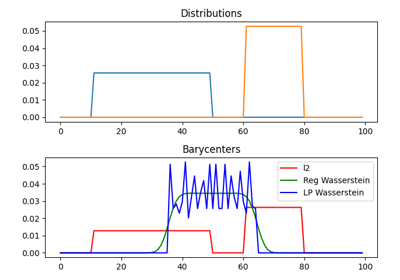1D Wasserstein barycenter: exact LP vs entropic regularization1D Wasserstein barycenter: exact LP vs entropic regularization

### Barycenters with free support

Estimating the Wasserstein barycenter with free support but fixed weights corresponds to solving the following optimization problem:

\begin{align}\begin{aligned}\min_{\{x_i\}} \quad \sum_{k} w_kW(\mu,\mu_k)\\s.t. \quad \mu=\sum_{i=1}^n a_i\delta_{x_i}\end{aligned}\end{align}

We provide a solver based on 20 in ot.lp.free_support_barycenter. This function minimize the problem and return a locally optimal support $$\{x_i\}$$ for uniform or given weights $$a$$.

#### Examples of free support barycenter estimation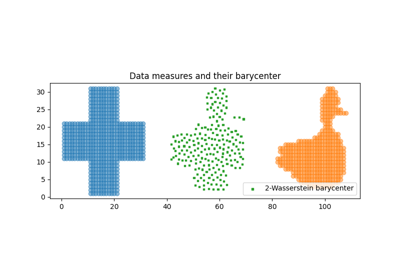2D free support Wasserstein barycenters of distributions

## Monge mapping and Domain adaptation

The original transport problem investigated by Gaspard Monge was seeking for a mapping function that maps (or transports) between a source and target distribution but that minimizes the transport loss. The existence and uniqueness of this optimal mapping is still an open problem in the general case but has been proven for smooth distributions by Brenier in his eponym theorem. We provide in ot.da several solvers for smooth Monge mapping estimation and domain adaptation from discrete distributions.

### Monge Mapping estimation

We now discuss several approaches that are implemented in POT to estimate or approximate a Monge mapping from finite distributions.

First note that when the source and target distributions are supposed to be Gaussian distributions, there exists a close form solution for the mapping and its an affine function 14 of the form $$T(x)=Ax+b$$ . In this case we provide the function ot.da.OT_mapping_linear that returns the operator $$A$$ and vector $$b$$. Note that if the number of samples is too small there is a parameter reg that provides a regularization for the covariance matrix estimation.

For a more general mapping estimation we also provide the barycentric mapping proposed in 6. It is implemented in the class ot.da.EMDTransport and other transport-based classes in ot.da . Those classes are discussed more in the following but follow an interface similar to scikit-learn classes. Finally a method proposed in 8 that estimates a continuous mapping approximating the barycentric mapping is provided in ot.da.joint_OT_mapping_linear for linear mapping and ot.da.joint_OT_mapping_kernel for non-linear mapping.

### Domain adaptation classes

The use of OT for domain adaptation (OTDA) has been first proposed in 5 that also introduced the group Lasso regularization. The main idea of OTDA is to estimate a mapping of the samples between source and target distributions which allows to transport labeled source samples onto the target distribution with no labels.

We provide several classes based on ot.da.BaseTransport that provide several OT and mapping estimations. The interface of those classes is similar to classifiers in scikit-learn. At initialization, several parameters such as regularization parameter value can be set. Then one needs to estimate the mapping with function ot.da.BaseTransport.fit. Finally one can map the samples from source to target with ot.da.BaseTransport.transform and from target to source with ot.da.BaseTransport.inverse_transform.

Here is an example for class ot.da.EMDTransport:

ot_emd = ot.da.EMDTransport()
ot_emd.fit(Xs=Xs, Xt=Xt)
Xs_mapped = ot_emd.transform(Xs=Xs)


A list of the provided implementation is given in the following note.

Note

Here is a list of the OT mapping classes inheriting from ot.da.BaseTransport

## Other applications

We discuss in the following several OT related problems and tools that has been proposed in the OT and machine learning community.

### Wasserstein Discriminant Analysis

Wasserstein Discriminant Analysis 11 is a generalization of Fisher Linear Discriminant Analysis that allows discrimination between classes that are not linearly separable. It consists in finding a linear projector optimizing the following criterion

$P = \text{arg}\min_P \frac{\sum_i OT_e(\mu_i\#P,\mu_i\#P)}{\sum_{i,j\neq i} OT_e(\mu_i\#P,\mu_j\#P)}$

where $$\#$$ is the push-forward operator, $$OT_e$$ is the entropic OT loss and $$\mu_i$$ is the distribution of samples from class $$i$$. $$P$$ is also constrained to be in the Stiefel manifold. WDA can be solved in POT using function ot.dr.wda. It requires to have installed pymanopt and autograd for manifold optimization and automatic differentiation respectively. Note that we also provide the Fisher discriminant estimator in ot.dr.fda for easy comparison.

Warning

Note that due to the hard dependency on pymanopt and autograd, ot.dr is not imported by default. If you want to use it you have to specifically import it with import ot.dr .

### Unbalanced optimal transport

Unbalanced OT is a relaxation of the entropy regularized OT problem where the violation of the constraint on the marginals is added to the objective of the optimization problem. The unbalanced OT metric between two unbalanced histograms a and b is defined as 25 10:

\begin{align}\begin{aligned}W_u(a, b) = \min_\gamma \quad \sum_{i,j}\gamma_{i,j}M_{i,j} + reg\cdot\Omega(\gamma) + reg_m KL(\gamma 1, a) + reg_m KL(\gamma^T 1, b)\\s.t. \quad \gamma\geq 0\end{aligned}\end{align}

where KL is the Kullback-Leibler divergence. This formulation allows for computing approximate mapping between distributions that do not have the same amount of mass. Interestingly the problem can be solved with a generalization of the Bregman projections algorithm 10. We provide a solver for unbalanced OT in ot.unbalanced. Computing the optimal transport plan or the transport cost is similar to the balanced case. The Sinkhorn-Knopp algorithm is implemented in ot.sinkhorn_unbalanced and ot.sinkhorn_unbalanced2 that return respectively the OT matrix and the value of the linear term.

Note

The main function to solve entropic regularized UOT is ot.sinkhorn_unbalanced. This function is a wrapper and the parameter method helps you select the actual algorithm used to solve the problem:

#### Examples of Unbalanced OT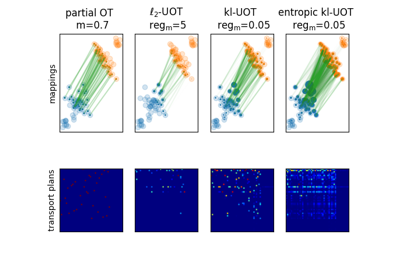2D examples of exact and entropic unbalanced optimal transport

### Unbalanced Barycenters

As with balanced distributions, we can define a barycenter of a set of histograms with different masses as a Fréchet Mean:

$\min_{\mu} \quad \sum_{k} w_kW_u(\mu,\mu_k)$

where $$W_u$$ is the unbalanced Wasserstein metric defined above. This problem can also be solved using generalized version of Sinkhorn’s algorithm and it is implemented the main function ot.barycenter_unbalanced.

Note

The main function to compute UOT barycenters is ot.barycenter_unbalanced. This function is a wrapper and the parameter method helps you select the actual algorithm used to solve the problem:

#### Examples of Unbalanced OT barycenters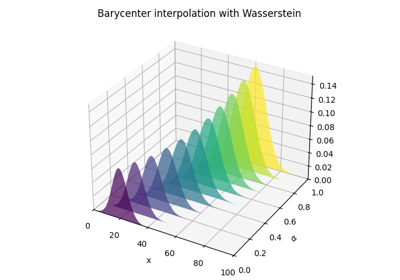1D Wasserstein barycenter demo for Unbalanced distributions

### Partial optimal transport

Partial OT is a variant of the optimal transport problem when only a fixed amount of mass m is to be transported. The partial OT metric between two histograms a and b is defined as 28:

\begin{align}\begin{aligned}\gamma = \arg\min_\gamma <\gamma,M>_F\\\begin{split}s.t. \gamma\geq 0 \\ \gamma 1 \leq a\\ \gamma^T 1 \leq b\\ 1^T \gamma^T 1 = m \leq \min\{\|a\|_1, \|b\|_1\}\end{split}\end{aligned}\end{align}

Interestingly the problem can be casted into a regular OT problem by adding reservoir points in which the surplus mass is sent 29. We provide a solver for partial OT in ot.partial. The exact resolution of the problem is computed in ot.partial.partial_wasserstein and ot.partial.partial_wasserstein2 that return respectively the OT matrix and the value of the linear term. The entropic solution of the problem is computed in ot.partial.entropic_partial_wasserstein (see 3).

The partial Gromov-Wasserstein formulation of the problem

\begin{align}\begin{aligned}GW = \min_\gamma \sum_{i,j,k,l} L(C1_{i,k},C2_{j,l})*\gamma_{i,j}*\gamma_{k,l}\\\begin{split}s.t. \gamma\geq 0 \\ \gamma 1 \leq a\\ \gamma^T 1 \leq b\\ 1^T \gamma^T 1 = m \leq \min\{\|a\|_1, \|b\|_1\}\end{split}\end{aligned}\end{align}

is computed in ot.partial.partial_gromov_wasserstein and in ot.partial.entropic_partial_gromov_wasserstein when considering the entropic regularization of the problem.

#### Examples of Partial OT2D examples of exact and entropic unbalanced optimal transport

### Gromov-Wasserstein

Gromov Wasserstein (GW) is a generalization of OT to distributions that do not lie in the same space 13. In this case one cannot compute distance between samples from the two distributions. 13 proposed instead to realign the metric spaces by computing a transport between distance matrices. The Gromow Wasserstein alignment between two distributions can be expressed as the one minimizing:

\begin{align}\begin{aligned}GW = \min_\gamma \sum_{i,j,k,l} L(C1_{i,k},C2_{j,l})*\gamma_{i,j}*\gamma_{k,l}\\s.t. \gamma 1 = a; \gamma^T 1= b; \gamma\geq 0\end{aligned}\end{align}

where :$$C1$$ is the distance matrix between samples in the source distribution and $$C2$$ the one between samples in the target, $$L(C1_{i,k},C2_{j,l})$$ is a measure of similarity between $$C1_{i,k}$$ and $$C2_{j,l}$$ often chosen as $$L(C1_{i,k},C2_{j,l})=\|C1_{i,k}-C2_{j,l}\|^2$$. The optimization problem above is a non-convex quadratic program but we provide a solver that finds a local minimum using conditional gradient in ot.gromov.gromov_wasserstein. There also exists an entropic regularized variant of GW that has been proposed in 12 and we provide an implementation of their algorithm in ot.gromov.entropic_gromov_wasserstein.

#### Examples of computation of GW, regularized G and FGW

Note that similarly to Wasserstein distance GW allows for the definition of GW barycenters that can be expressed as

$\min_{C\geq 0} \quad \sum_{k} w_k GW(C,Ck)$

where $$Ck$$ is the distance matrix between samples in distribution $$k$$. Note that interestingly the barycenter is defined as a symmetric positive matrix. We provide a block coordinate optimization procedure in ot.gromov.gromov_barycenters and ot.gromov.entropic_gromov_barycenters for non-regularized and regularized barycenters respectively.

Finally note that recently a fusion between Wasserstein and GW, coined Fused Gromov-Wasserstein (FGW) has been proposed 24. It allows to compute a similarity between objects that are only partly in the same space. As such it can be used to measure similarity between labeled graphs for instance and also provide computable barycenters. The implementations of FGW and FGW barycenter is provided in functions ot.gromov.fused_gromov_wasserstein and ot.gromov.fgw_barycenters.

## Solving OT with Multiple backends on CPU/GPU

Since version 0.8, POT provides a backend that allows to code solvers independently from the type of the input arrays. The idea is to provide the user with a package that works seamlessly and returns a solution for instance as a Pytorch tensors when the function has Pytorch tensors as input.

### How it works

The aim of the backend is to use the same function independently of the type of the input arrays.

For instance when executing the following code

# a and b are 1D histograms (sum to 1 and positive)
# M is the ground cost matrix
T = ot.emd(a, b, M)  # exact linear program
w = ot.emd2(a, b, M)  # Wasserstein computation


the functions ot.emd and ot.emd2 can take inputs of the type numpy.array, torch.tensor or jax.numpy.array. The output of the function will be the same type as the inputs and on the same device. When possible all computations are done on the same device and also when possible the output will be differentiable with respect to the input of the function.

### GPU acceleration

The backends provide automatic computations/compatibility on GPU for most of the POT functions. Note that all solvers relying on the exact OT solver en C++ will need to solve the problem on CPU which can incur some memory copy overhead and be far from optimal when all other computations are done on GPU. They will still work on array on GPU since the copy is done automatically.

Some of the functions that rely on the exact C++ solver are:

### List of compatible Backends

• Numpy (all functions and solvers)

• Pytorch (all outputs differentiable w.r.t. inputs)

• Jax (Some functions are differentiable some require a wrapper)

• Tensorflow (all outputs differentiable w.r.t. inputs)

• Cupy (no differentiation, GPU only)

### List of compatible modules

This list will get longer for new releases and will hopefully disappear when POT become fully implemented with the backend.

## FAQ

1. How to solve a discrete optimal transport problem ?

The solver for discrete OT is the function ot.emd that returns the OT transport matrix. If you want to solve a regularized OT you can use ot.sinkhorn.

Here is a simple use case:

# a and b are 1D histograms (sum to 1 and positive)
# M is the ground cost matrix
T = ot.emd(a, b, M)  # exact linear program
T_reg = ot.sinkhorn(a, b, M, reg)  # entropic regularized OT


More detailed examples can be seen on this example: Optimal Transport between 2D empirical distributions

2. pip install POT fails with error : ImportError: No module named Cython.Build

As discussed shortly in the README file. POT<0.8 requires to have numpy and cython installed to build. This corner case is not yet handled by pip and for now you need to install both library prior to installing POT.

Note that this problem do not occur when using conda-forge since the packages there are pre-compiled.

See Issue #59 for more details.

3. Why is Sinkhorn slower than EMD ?

This might come from the choice of the regularization term. The speed of convergence of Sinkhorn depends directly on this term 22. When the regularization gets very small the problem tries to approximate the exact OT which leads to slow convergence in addition to numerical problems. In other words, for large regularization Sinkhorn will be very fast to converge, for small regularization (when you need an OT matrix close to the true OT), it might be quicker to use the EMD solver.

Also note that the numpy implementation of Sinkhorn can use parallel computation depending on the configuration of your system, yet very important speedup can be obtained by using a GPU implementation since all operations are matrix/vector products.